International Journal of Discrete Mathematics
Volume 2, Issue 1, March 2017, Pages: 17-19

Fixed Point Theorem in Cone Metric Space

K. Prudhvi

Department of Mathematics, University College of Science, Saifabad, Osmania University, Hyderabad, IndiaK. Prudhvi. Fixed Point Theorem in Cone Metric Space. International Journal of Discrete Mathematics. Vol. 2, No. 1, 2017, pp. 17-19. doi: 10.11648/j.dmath.20170201.14

Received: December 19, 2016; Accepted: January 24, 2017; Published: February 22, 2017

Abstract: In this paper, we prove a unique common fixed point theorem for four self-mappings in cone metric spaces by using the continuity and commuting mappings. Our result extends,improvesthe results of [M. Abbas and G. Jungck, Common fixed point results for non commuting mappings without continuity in cone metric spaces, J. Math. Anal. Appl. 341(2008) 416-420].

Keywords: Cone Metric Space, Fixed Point, Common Fixed Point

Contents

1. Introduction and Preliminaries

In 2007, Huang and Zhang  introduced the concept of a cone metric space, and they replacing the set of real numbers by an ordered Banach space and proved some fixed point theorems for mappings satisfying the different contractive conditions with using the normality condition in cone metric spaces. Subsequently many authors were inspired with these results and they (see, e.g., [2-4, 6, 8, 9, 10]) extended, improved and generalized the fixed point theorems of Huang and Zhang . In 2008, Abbas and Jungck  obtained some fixed point theorems in cone metric spaces. In this paper, we proved a unique common fixed point theorem for four self-mappings in cone metric spaces using the continuity and commuting mappings. This result extends, improves and generalizes the results of Abbas and Jungck .

The following definitions and properties are in .

Definition 1.1 . Let B be a real Banach space and P be a subset of B. The set P is called a cone if and only if:

(a). P is closed, non–emptyand P ≠ {0};

(b). a, b∈ℝ, a,b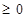, x, yP implies ax+byP;

(c). xP and -xP implies x = 0.

Definition 1.2 . Let P be a cone in a Banach space B, define partial ordering ‘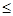’ with respect to P by xy if and only if y-xP. We shall write x<y to indicate x≤y but x ≠ ywhile x<<y will stand for y-xint P, where int P denotes the interior of the set P. This cone P is called an order cone.

Definition 1.3 . Let B be a Banach space and P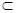B be an order cone. The order cone P is called normal if there exists M>0 such that for all x, yB,

0 ≤ x ≤y implies ║x║≤ M║y║.

The least positive number M satisfying the above inequality is called the normal constant of P.

Definition 1.4 . Let X be a nonempty set of Banach space B. Suppose that the map d: X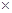X→ B satisfies:

(a). 0 ≤ d(x, y) for all x, yX and d(x,y) = 0 if and only if

x = y;

(b). d(x, y) = d(y, x) for all x, yX;

(c). d(x, y)d(x, z)+d(z, y) for all x, y, zX.

Then d is called a cone metric on X and (X, d) is called a cone metric space.

It is clear that the concept of a cone metric space is more general than that of a metric space.

Example 1.5 . Let B =2, P = {(x, y)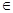B such that : x, y  0}2, X =and d: X × X→B such that d(x, y) = (│x - y│, α│x - y│), where α ≥ 0 is a constant. Then (X, d) is a cone metric space.

Definition 1.6 . Let (X, d) be a cone metric space. We say that {xn} is

(i) a Cauchy sequence if for every c in B with c>>0, there is a natural number N such that for all

n, m>N, d(xn, xm)<<c;

(ii) a convergent sequence if for any c>>0, there is a natural number N such thatfor all n>N, d(xn, x) <<c, for some fixed x in X. We denote this xn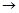x (as n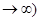.

Lemma 1.7 . Let (X, d) be a cone metric space. Let {xn} be a sequence in X. Then {xn} is a Cauchy sequence if and only if d(xn, xm)®0 as n, m®∞.

In 2008 Abbas and Jungck  proved the following Theorem without continuity.

Theorem 1.8. (Theorem 2.1 of ) Let (X, d) be a complete cone metric space and P be normal cone with normal constant K. Suppose mappings f,g:XX satisfy

d(fx, fy) ≤ kd(gx, gy)

for all x, yÎX, where k[0,1) is a constant. If the range of g contains the range of f g(X) is complete subspace of X, then f and g have a unique coincidence point in X. Moreover, if f and g weakly compatible, f and g have a unique common fixed point.

We have extended and generalized the above Theorem for four self-mappings using the continuity and commuting mappings.

2. Main Result

In this section, we prove a unique common fixed point theorem for four self-mappings in cone metric spaces by using the continuity and commuting mappings.

The following theorem is extended and generalized the Theorem 2.1 of .

Theorem 2.1. Let (X, d) be a complete cone metric space. And P be normal cone with normal constant M. Suppose that the four self-mappings S and I, T and J are commuting mappings satisfying the following

d(Sx, Ty) ≤ ld(Ix, Jy)                               (1)

for all x, yÎX,where 0<l<1. If S(X) Ì J(X) and T(X) Ì I(X) and if I and J are continuous, then all S, T, I and J have a unique common fixed point.

Proof: Let x0ÎX be arbitrary. Since S(X)Ì J(X), let x1ÎX be such that Jx1 = Sx0, also, as Tx1ÎI(X), letx2ÎX be such that Ix2 = Tx1. In general, x2n+1ÎX is chosen such that

Jx2n+1 = Sx2nandx2n+2ÎX is chosen such that

Ix2n+2=Tx2n+1; n = 0, 1, 2, 3, we denote

y2n=Jx2n+1 = Sx2n,

y2n+1=Ix2n+2 = Tx2n+1, n = 0, 1, 2, 3,...

We shall show that {yn} is a Cauchy sequence. For this we have

d(y2n,y2n+1) = d(Sx2n, Tx2n+1)ld(Ix2n,Jx2n+1) =ld(y2n-1, y2n)

d(y2n,y2n-1) = d(Sx2n, Tx2n-1)ld(Ix2n,Jx2n-1) = ld(y2n-1, y2n-2),

that is, for n ≥ 2

d(yn, yn -1)ld(yn -1, yn-2).

Hence, for n ≥ 2 it follows that

d(yn,yn-1) ≤ … ≤ln-1 d(y1, y0).

By the triangle inequality, for n>m we have

d(yn, ym) ≤ d(yn,yn-1) +… + d(ym+1, ym),

≤ (ln-1 +ln-1 +…+lm)d(y1, y0),

lm/1-ld(y1, y0).

By (Definition 1.3) we haved(yn,ym)≤ M(lm /1-l)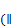d(y1,y0))) (as m®∞)

By the Lemma 1.7, then {yn} is a Cauchy sequence in X.

Let zÎX be such that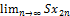==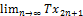=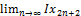= z.

Since, I is continuous and S and I commute, it follows that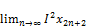= Iz,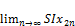=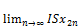= Iz.

From (1), it follows that

d(SIx2n, Tx2n+1)ld(I2x2n, Jx2n+1).

Letting n®∞, we get that

d(Iz, z) ≤ ld(Iz, z).

That is, as 0 <l< 1 it follows that Iz = z.

Similarly, since J is continuous and T and J commute, it follows that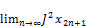= Jz,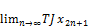=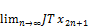= Jz.

From (1), it follows that

d(Sx2n, TJx2n+1)ld(Ix2n, J2 x2n+1).

Letting n®∞, we get that

d(z, Jz) ≤ ld(z, Jz),

that is again it follows that z = Tz.

From (1) we have Iz = z.

d(Sz, Tx2n+1)ld(z, Jx2n+1).

Letting n®∞, we get that

d(Sz, z) = 0. That is, Sz = z.

Again (1) we have d(Sz, Tz) = 0, that is Sz = Tz.

Thus, we proved that Sz = Tz = Iz = Jz = z.

Let wbe another common fixed point in X of S, T, I and J, then

d(z, w) = d(Sz, Tw) ≤ld(z, w).

Since 0<l<1, it follows that d(z,w) = 0.That is z = w.

Therefore, z is a unique common fixed point of S, T, I and J.

This completes the proof.

Remark 2.2. If we choose S = T = f and I = J = g and without continuity, non-commuting mappings in the above Theorem 2.1, then we get the Theorem 2.1 of .

3.Conclusion

In this paper, the author extended, improved and generalized the Theorem 2.1 of [M. Abbas and G. Jungck, Common fixed point results for non commuting mappings without continuity in cone metric spaces, J. Math. Anal. Appl. 341(2008) 416-420] , for four self-mappings with using the continuity, and commuting mappings. In the Theorem 2.1 of  the authors Abbas and Jungck  have proved fixed point theorem for two self-mappings without using the continuity.

References

1. M. Abbas and G. Jungck, Common fixed point results for non commuting mappings without continuity in cone metric spaces, J. Math. Anal. Appl. 341(2008) 416-420.
2. M. Abbas, B. E. Rhoades, Fixed and periodic point results in cone metric spaces, Appl. Math. Lett. 21(2008)511-515.
3. I. Altun, B. Damnjanovic, D. Djoric, Fixed point and common fixed point theorems on ordered cone metric spaces, Appl. Math. Lett. (2009)doi:10.1016/j.aml.2009.09.016.
4. M. Amari, D.El Moutawakil, Some new common fixed point theorems under strict contractive conditions, J. Math. Anal. Appl. 270(2002)181-188.
5. M. Arshad, A. Azam, P. Vetro, Some common fixed point results on cone metric spaces, Fixed Point Theory Appl. (2009)11, Article ID 493965.
6. Guangxing Song, Xiaoyan Sun, Yian Zhao, Guotao Wang, New common fixed point theorems for maps on cone metric spaces, Appl. Math. Lett. 32(2010)1033-1037.
7. L.G.Huang, X.Zhang,Cone metric spaces and fixed point theorems of contractive mappings, J. Math. Anal. Appl. 332(2) (2007)1468-1476.
8. D. IIic, V.Rakocevic, Common fixed points for maps on cone metric space, J. Math. Anal. Appl. 341(2008) 876-886.
9. S. Radenović, B.E. Rhoades, Fixed point theorem for two non-self mappings in cone metric spaces, Comput. Math. Appl. 57 (2009) 1701–1707.
10. Sh. Rezapour and R. Hamlbarani, Some notes on the paper cone metric spaces and fixed point theorem of contractive mappings, J. Math. Anal. Appl. 345(2008),719-724.

 Contents 1. 2. 3.
Article ToolsAbstractPDF(149K)